×#### Thank you for registering.

One of our academic counsellors will contact you within 1 working day.

Click to Chat

1800-1023-196

+91-120-4616500

CART 0

• 0

MY CART (5)

Use Coupon: CART20 and get 20% off on all online Study Material

ITEM
DETAILS
MRP
DISCOUNT
FINAL PRICE
Total Price: Rs.

There are no items in this cart.
Continue Shopping• Complete JEE Main/Advanced Course and Test Series
• OFFERED PRICE: Rs. 15,900
• View Details

```Revision Notes on Introduction to Graphs

Graph

The graphs are the visual representation of the data collected in numerical form so that it could be easily understood. Graphs are generally used for comparisons between two or more data.

Types of Graph

1. Bar Graph

A bar graph is a type of graph which is used to show comparisons between different categories. In this type of graph, bars of uniform width are used to represent the different quantities and their height is proportional to their respective values.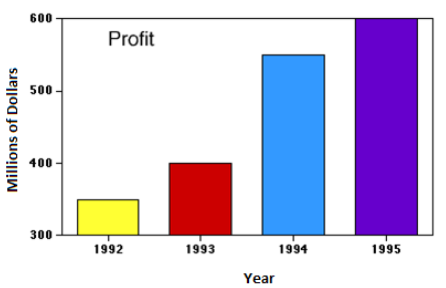This is the bar graph which shows the profit of the company over different years.

2. Double Bar Graph

This is the same as the bar graph but two types of data are shown here simultaneously. Generally, this type of graph is used to show a comparison between two data.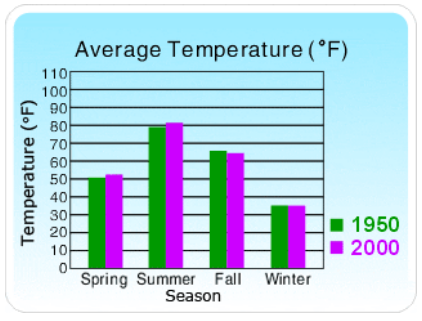This Double bar graph shows the average temperature in different seasons in two different years. Green bars show the temperature in 1950 and pink bars show the temperature in 2000. Hence we can easily compare the up and downs in average temperature from 1950 to 2000.

3. A Pie Chart (Circle Graph)

When we represent the given data in the circular form then it is said to be a pie chart. It shows the part of a whole.This pie chart shows the percentage of people watching different kind of movies. From this chart, we can easily get that the maximum number of people like romantic movies and the least number of people like drama movies.

4. Histogram

When we have grouped data in the form of class intervals then we can draw a histogram. It is also a bar graph but there is no gap between the bars as it is a graph of continuous data.This histogram shows the distribution of people in a society according to their age.

5. Line Graph

When we need to see the changes continuously over a period of time then we use a line graph.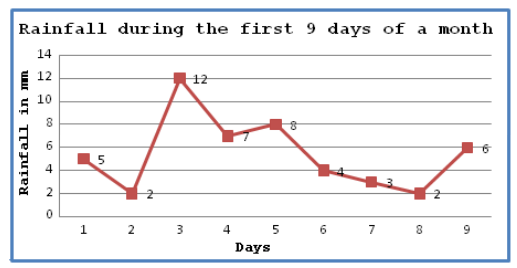Here the horizontal line (x-axis) shows the number of days and vertical line (y-axis) shows the rainfall in mm on every successive day.

By this line graph, we can easily understand the changes in rainfall during these 9 days of a month.

Linear Graphs

A line graph which is a whole unbroken line is called a Linear Graph.

1. Location of a Point

For making a linear graph we use the Cartesian plane. It is that system on which we mark the points with the help of Vertical and Horizontal Lines.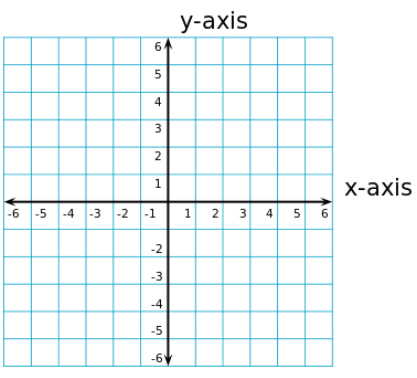This is a Cartesian plane which is like a square grid sheet. We make a horizontal line (x-axis) and a vertical line (y-axis) which divides it into four quadrants.

2. Coordinates of a Point

To write the coordinates of a point we need an x - coordinate and a y-coordinate of a point.

x-coordinate tells how many units to move right or left. It is also called the Abscissa.

y-coordinate tells how many units to move up or down. It is also called the Ordinate.

While writing the coordinates of a point in the coordinate plane, the x - coordinate comes first, and then the y - coordinate. We place the coordinates in brackets.

Coordinates of the point of intersection of x-axis and y-axis is (0, 0).this is called Origin.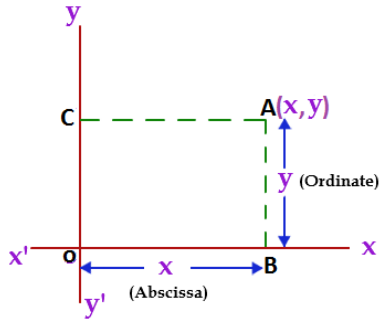In the above figure, OB = CA = x coordinate (Abscissa), and CO = AB = y coordinate (Ordinate).

We write the coordinate as (x, y).

Example

Plot the given points on the graph sheet.

(2, 3)

(-3, 1)

(-1.5, – 2.5)

Solution: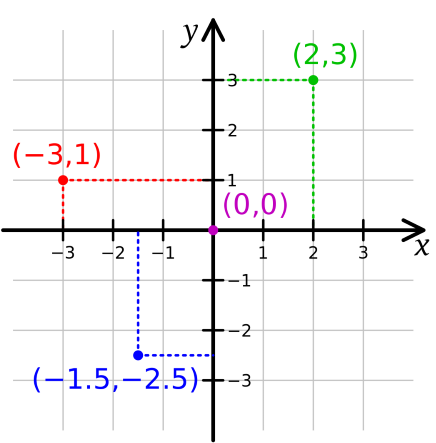Remark: If x ≠ y, then (x, y) ≠ (y, x), and (x, y) = (y, x), if x = y.

Some Applications of Graph

In our day to day life, there are so many situations where we can use the graph for comparisons and analysis.

1. Independent Variable

Anything which is completely independent and its movement do not depend on any other factor then it is called Independent Variable.

2. Dependent Variable

Anything which increases or decreases with the movement of any other factor or it is dependent on any other factor then it is called Dependent Variable.

The Relationship between the Independent and Dependent VariableThe above graph shows that the revenue will increase with the increase in the number of cars washed. So the revenue is the dependent variable and the number of cars washed is an independent variable.
```### Course Features

• 728 Video Lectures
• Revision Notes
• Previous Year Papers
• Mind Map
• Study Planner
• NCERT Solutions
• Discussion Forum
• Test paper with Video Solution